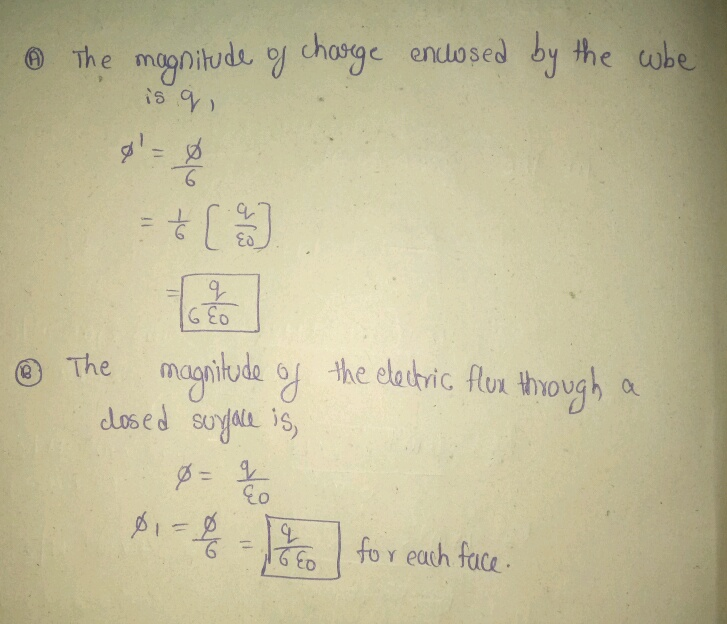# A point charge of magnitude q is at the center of a cube with sides of length l.

10. A point charge of magnitude q is at the center of a
cube with sides of length L. q L A. What is the electric flux Φ through each of the six faces of
the cube? Use ϵ0 for the permittivity of free space. ϵ B. What would be the flux Φ1 through a face of the cube if its
sides were of length L1? L Use ϵ0 for the permittivity of free space.
ϵ